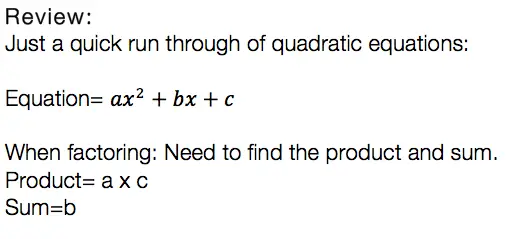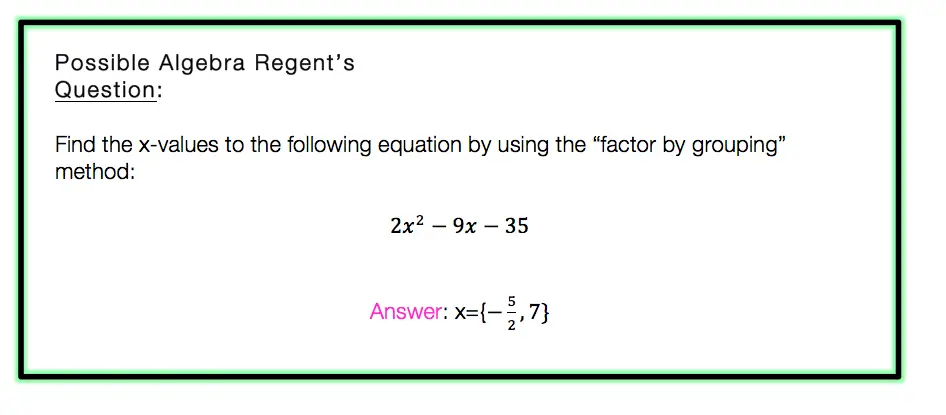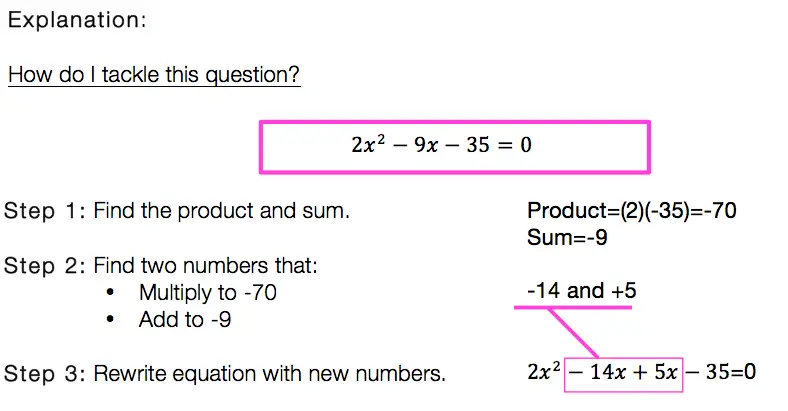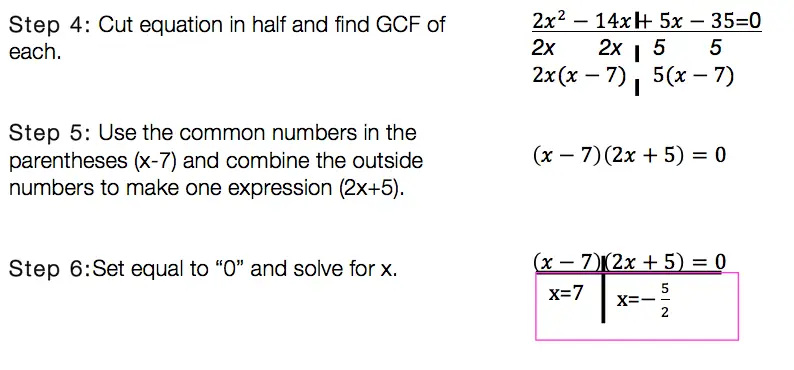# Blog

## Factor by Grouping: Algebra 2/Trig.

Hey math friends! In this post, we are going to go over Factor by Grouping, one of the many methods for factoring a quadratic equation.  There are so many methods to factor quadratic equations, but this is a great choice, for when a is greater than 1. Also, if you need to review different types of factoring methods, just check out this link here.  Stay curious and happy calculating! 🙂 Before we go any further, let’s just take a quick look at what a quadratic equation is:Usually, we can just find the products, the sum, re-write the equation, solve for x, and be on our merry way.  But if you notice, there is something special about the question below. The coefficient “a” is greater than 1/. This is where factor by grouping comes in handy!Now that we know why and when we need to factor by grouping lets take a look at our Example:Factor By Grouping: Hard to solve? No.  Hard to remember? It can be, just remember to practice, practice practice! Also, if you are in need of a review of other methods of factoring quadratic equations, click this link here.

Want more Mathsux?  Don’t forget to check out our Youtube channel and more below! And if you have any questions, please don’t hesitate to comment. Happy Calculating! 🙂

## Equation of A Circle: Geometry

Equation of A Circle

Ahoy math friends and welcome to Math Sux! In this post, we are going to learn about the equation of a circle, mainly how to write it when given a circle on a coordinate plane. We’ll see how to find the radius and center from the graphed circle and then see how to transfer that into our equation of a circle. And we’re going to do all of this step by step with the following Regents question. Stay curious and happy calculating! 🙂

## How do I Answer this Question?

Let’s mark up this coordinate plane and take as much information away from it as possible. When looking at this coordinate plane, we can find the value of the circle’s center and the circle’s radius.

Now that found the center and radius, we can fit each into our equation:

Center=(-3,-4)

The only equation that matches our center and radius, is choice (2).

If the above answer makes sense to you, that’s great! If you need a little further explanation, keep going!

## Equation of a Circle:

Equation of a Circle: Let’s take a look at our answer and break down what each part means:

So what do you think of circles now?  Not to shabby, ehh?  🙂

Looking for more on circles?  Check out this post on how to find the Area of a Sector here!

## Recursive Formula: Algebra

Howdy math peeps! In this post, we are going to go over the recursive formula step by step by reviewing a Regent’s question. Yes, it is the recursive formula jam, well at least it’s my kind of jam.  These things may look weird, confusing, and like a “what am I doing?” moment, but trust me they are no so bad!

How do I answer this question?

At first glance, all of these answer choices may look exactly the same. The first thing I would want to do with this question is to identify how all of theses answer choices are different. Take a look below:

What is the Recursive Formula?

A Recursive Formula is a formula that forms a sequence based on the previous term value. All this means is that it uses a formula to form a sequence-based pattern.

Let’s go through each choice to identify the answer:

Our goal is to test out each choice given, until we get the desired sequence:

Still have questions about recursive formulas? Check out more on recursive formulas here!

Still got questions? No problem! Check out the video above for more or try the NYS Regents question below, and please don’t hesitate to comment with any questions. Happy calculating! 🙂

## Solving Log Equations: Algebra 2/Trig.

Ahoy math friends! In this post, we are going to focus on solving log equations by solving this Regents questions step by step. We’ll answer this question right away! But if you need more of a review, keep reading and you will find what logarithms are, the different kinds of logarithms rules, and some simpler examples. Ready for our first example?! Check it out below:

## How do I Answer this Question?

Step 1: Let’s re-write the equation to get rid of the “log.”

Step 2: Solve for x in our new equation (5x-1)(1/3)=4

If the above answer makes sense to you, great! If not, that’s ok too, keep reading for a review on solving log equations.

## What are Logarithms?

Logarithms are inverses of exponential equations. Take a look below for a clearer picture.

Basic Formula:

Logarithms can be re-written to get rid of the word “log.” This makes them easier to solve and understand.

Log Rules:

There are a few rules you have to memorize get used to with practice. These rules are used when solving for x in different kinds of algebraic log problems:

Still got questions?  No problem! Check out the videos below and the post here for more on logarithms! Also don’t forget to subscribe below to get the latest FREE math videos, lessons, and practice questions from MathSux. Thanks for stopping by and happy calculating! 🙂